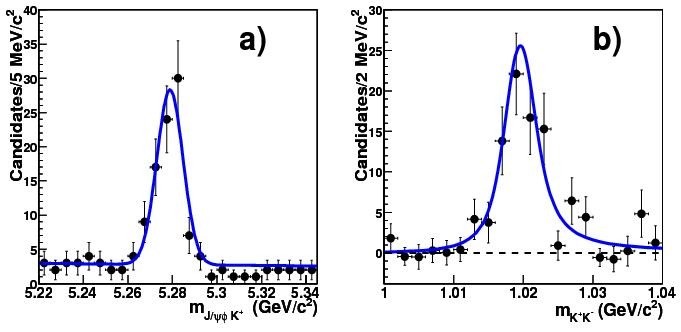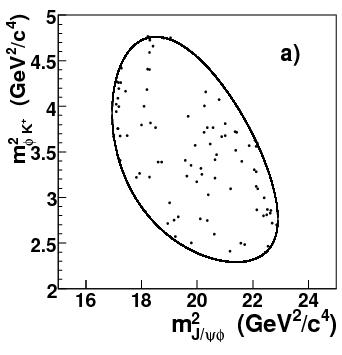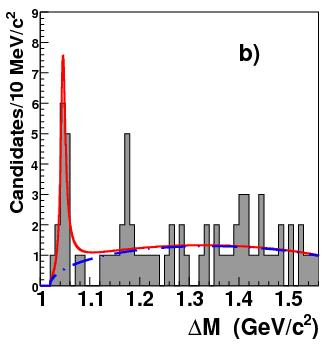## Streaming video for Y(4140) discoveryMarch 17, 2009

Posted by dorigo in news, physics, science.
Tags: , , , , ,

The CDF collaboration will present at a public venue (Fermilab’s Wilson Hall) its discovery of the new Y(4140) hadron, a mysterious particle created in B meson decays, and observed to decay strongly into a$J/\psi \phi$ state, a pair of vector mesons. I have described that exciting discovery in a recent post.

From this site you can connect to streaming video (starting at 4.00PM CDT, or 9.00PM GMT – should last about 1.30 hours).

## CDF discovers a new hadron!March 13, 2009

Posted by dorigo in news, physics, science.
Tags: , , , ,

This morning CDF released the results of a search for narrow resonances produced in B meson decays, and in turn decaying into a pair of vector mesons: namely,$Y \to J/\psi \phi$. This Y state is a new particle whose exact composition is as of yet unknown, except that CDF has measured its mass (4144 MeV) and established that its decay appears to be mediated by strong interactions, given that the natural width of the state is in the range of a few MeV. I describe succintly the analysis below, but first let me make a few points on the relevance of area of investigation.

Heavy meson spectroscopy appears to be a really entertaining research field these days. While all eyes are pointed at the searches for the Higgs boson and supersymmetric particles, if not at even more exotic high-mass objects, and while careers are made and unmade on those uneventful searches, it is elsewhere that action develops. Just think about it: the$\Xi_b$ baryon, the$\Omega_b$, those mysterious X and Y states which are still unknown in their quark composition. Such discoveries tell the tale of a very prolific research field: one where there is really a lot to understand.

Low-energy QCD  is still poorly known and not easily calculable. In frontier High-Energy Physics we bypassed the problem for the sake of studying high-energy phenomena by tuning our simulations such that their output well resembles the result of low-energy QCD processes in all cases where we need them -such as the details of parton fragmentation, or jet production, or transverse momentum effects in the production of massive bodies. However, we have not learnt much with our parametrizations:  those describe well what we already know, but they do not even come close to guessing whatever we do not know. Our understanding of low-energy QCD is starting to be a limiting factor in cosmological studies, such as in baryogenesis predictions. So by all means, let us pursue low-energy QCD in all the dirty corners of our produced datasets at hadron colliders!

CDF is actively pursuing this task. The outstanding spectroscopic capabilities of the detector, combined with the huge size of the dataset collected since 2002, allow searches for decays in the one-in-a-million range of branching ratios. The new discovery I am discussing today has indeed been made possible by pushing to the limit our search range.

The full decay chain which has been observed is the following:$B^+ \to Y K^+ \to J/\psi \phi K^+ \to \mu^+ \mu^- K^+ K^- K^+$. That$J/\psi$ mesons decay to muon pairs is not a surprise, as is the decay to two charged kaons of the$\phi$ vector meson. Also the original decay of the B hadron into the$J/\psi \phi K$ final state is not new: it had been in fact observed previously. What had not been realized yet, because of the insufficient statistics and mass resolution, is that the$J/\psi$ and$\phi$ mesons produced in that reaction often “resonate” at a very definite mass value, indicating that in those instances the$B \to J/\psi \phi K$ decay actually takes place in two steps as the chain of two two-body decays:$B \to Y K$ and$Y \to J/\psi \phi$.

The new analysis by CDF is a pleasure to examine, because the already excellent momentum resolution of the charged particle tracking system gets boosted when constraints are placed on the combined mass of multi-body systems. Take the B meson, reconstructed with two muons and three charged tracks, each assumed to be a kaon: if you did not know that the muon pair comes from a$J/\psi$ nor that two of the kaons come from a$\phi$, the mass resolution of the system would be in the few tens of MeV range. Instead, by forcing the momenta of the two muons to be consistent with the World average mass of the$J/\psi$,$M_{J/\psi}=3096.916 \pm 0.011 MeV$ , and by imposing that the two kaons make exactly the extremely well-known$\phi$ mass ($M_\phi=1019.455 \pm 0.020 MeV$), much of the uncertainty on the daughter particle momenta disappears, and the B meson becomes an extremely narrow signal: its mass resolution is just 5.9 MeV, a per-mille measurement event-by-event!The selection of signal events requires several cleanup cuts, including mass window cuts around the J/Psi and phi masses, a decay length of the reconstructed B+ meson longer than 500 microns, and a cut on the log-likelihood ratio fed with dE/dx and time-of-flight information capable of discriminating kaon tracks from other hadrons. After those cuts, the B+ signal really stands above the flat background. There is a total of 78+-10 events in the sample after these cuts, and this is the largest sample of such decays ever isolated. It is shown above (left), together with the corresponding distribution in the$\phi \to KK$ candidate mass (right).A Dalitz plot of the reconstructed decay candidates is shown in the figure on the right. A Dalitz plot is a scatterplot of the squared invariant mass of a subset of the particles emitted in the decay, versus the squared invariant mass of another subset. If the decay proceeds via the creation of an intermediate state, one may observe a horizontal or vertical cluster of events. Judge by yourself: do the points appear to spread evenly in the allowed phase space of the B+ decays ?

The answer is no: a significant structure is seen corresponding to a definite mass of the$J/\psi \phi$ system. A histogram of the difference between the reconstructed mass of the$J/\psi \phi$ system and the$J/\psi$ mass is shown in the plot below: a near-threshold structure appears at just above 1 GeV energy. An unbinned fit to a relativistic Breit-Wigner signal shape on top of the expected background shape shows a signal at a mass difference of$\Delta M=1046.3 \pm 2.9 MeV$, with a width of 11.7+-5.7 MeV.The significance of the signal is, after taking account of trial factors, equal to 3.8 standard deviations. For the non-zero width hypothesis, the significance is of 3.4 standard deviations, implying that the newfound structure has strong decay. The mass of the new state is thus of 4143+-2.9 MeV.

The new state is above the threshold for decay to pair of charmed hadrons. The decay of the state appears to occur to a pair of vector mesons,$J/\psi \phi$, in close similarity to a previous state found at 3930 MeV, the Y(3930), which also decays to two vector mesons in$Y \to J/\psi \omega$. Therefore, the new state can be also called a Y(4140).

Although the significance of this new signal has not reached the coveted threshold of 5 standard deviations, there are few doubts about its nature. Being a die-hard sceptic, I did doubt about the reality of the signal shown above for a while when I first saw it, but I must admit that the analysis was really done with a lot of care. Besides, CDF now has tens of thousands of fully reconstructed B meson decays available, with which it is possible to study and understand even the most insignificant nuances to every effect, including reconstruction problems, fit method, track characteristics, kinematical biases, you name it. So I am bound to congratulate with the authors of this nice new analysis, which shows once more how the CDF experiment is producing star new results not just in the high-energy frontier, but as well as in low-energy spectroscopy. Well done, CDF!